Xavier1596265944

• 8

获得赞
• 4

发布的文章
• 0

答辩的项目

## Fast-SCNN的解释以及代码的实现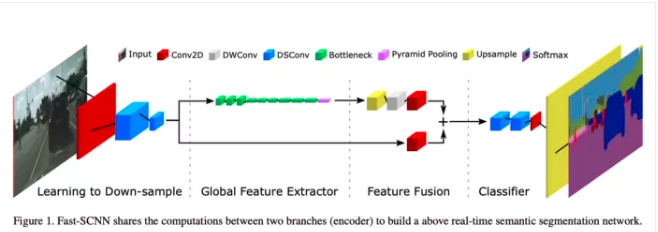Fast Segmentation Convolutional Neural Network (Fast- scnn)是一种针对高分辨率图像数据的实时语义分割模型，适用于低内存嵌入式设备上的高效计算。原论文的作者是：Rudra PK Poudel, Stephan Liwicki and Roberto Cipolla。本文中使用的代码并不是作者的正式实现，而是我对论文中描述的模型的重构的尝试。

Fast-SCNN的一些关键方面是：

1. 在高分辨率图像(1024 x 2048px)上的实时分割
2. 得到准确率为68%的平均IOU
3. 在Cityscapes数据集上每秒处理123.5帧
4. 不需要大量的预训练
5. 结合高分辨率的空间细节和低分辨率提取的深度特征

1. 学习下采样
2. 全局特征提取器
3. 特征融合
4. 分类器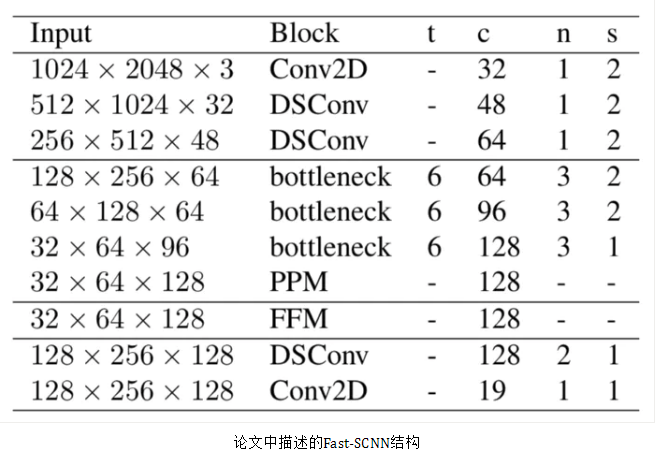1. 学习下采样

学习下采样模块使用3层来提取这些全局特征。分别是：Conv2D层，然后是2个深度可分离的卷积层。在实现过程中，在每个Conv2D和深度可分离的Conv层之后，使用一个Batchnorm层和Relu激活，因为通常在这些层之后引入Batchnorm和激活是一种标准实践。这里，所有3个层都使用2的stride和3x3的内核大小。

`pip install tensorflow-gpu==2.0.0`

`import tensorflow as tf`

`input_layer = tf.keras.layers.Input(shape=(2048, 1024, 3), name = 'input_layer')`

```def conv_block(inputs, conv_type, kernel, kernel_size, strides, padding='same', relu=True):

if(conv_type == 'ds'):
else:

x = tf.keras.layers.BatchNormalization()(x)

if (relu):
x = tf.keras.activations.relu(x)

return x```

```lds_layer = conv_block(input_layer, 'conv', 32, (3, 3), strides = (2, 2))
lds_layer = conv_block(lds_layer, 'ds', 48, (3, 3), strides = (2, 2))
lds_layer = conv_block(lds_layer, 'ds', 64, (3, 3), strides = (2, 2))```

2. 全局特征提取器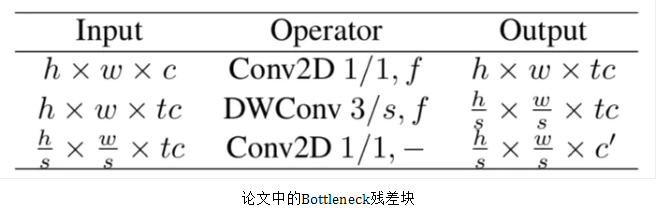以上是本文对bottleneck残差块的描述。与上面类似，现在让我们使用tf.keras高级api来实现。

```def _res_bottleneck(inputs, filters, kernel, t, s, r=False):

tchannel = tf.keras.backend.int_shape(inputs)[-1] * t

x = conv_block(inputs, 'conv', tchannel, (1, 1), strides=(1, 1))

x = tf.keras.layers.DepthwiseConv2D(kernel, strides=(s, s), depth_multiplier=1, padding='same')(x)
x = tf.keras.layers.BatchNormalization()(x)
x = tf.keras.activations.relu(x)

x = conv_block(x, 'conv', filters, (1, 1), strides=(1, 1), padding='same', relu=False)

if r:
return x```

```![1_xO4huN3z718VyT6fG73PjQ](Fast-SCNN explained and implemented using Tensorflow 2.0.assets/1_xO4huN3z718VyT6fG73PjQ.png)def bottleneck_block(inputs, filters, kernel, t, strides, n):
x = _res_bottleneck(inputs, filters, kernel, t, strides)

for i in range(1, n):
x = _res_bottleneck(x, filters, kernel, t, 1, True)```

```gfe_layer = bottleneck_block(lds_layer, 64, (3, 3), t=6, strides=2, n=3)
gfe_layer = bottleneck_block(gfe_layer, 96, (3, 3), t=6, strides=2, n=3)
gfe_layer = bottleneck_block(gfe_layer, 128, (3, 3), t=6, strides=1, n=3)```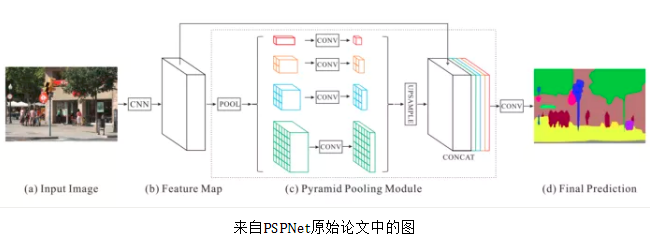PPM使用上个卷积层出来的特征图，然后应用多个子区域平均池化和以及上采样函数来得到不同的子区域的特征表示，然后连接在一起，这样就带有了本地和全局上下文的信息，可以让图像的分割过程更准确。

```def pyramid_pooling_block(input_tensor, bin_sizes):
concat_list = [input_tensor]
w = 64
h = 32

for bin_size in bin_sizes:
x = tf.keras.layers.AveragePooling2D(pool_size=(w//bin_size, h//bin_size), strides=(w//bin_size, h//bin_size))(input_tensor)
x = tf.keras.layers.Conv2D(128, 3, 2, padding='same')(x)
x = tf.keras.layers.Lambda(lambda x: tf.image.resize(x, (w,h)))(x)

concat_list.append(x)

return tf.keras.layers.concatenate(concat_list)```

`gfe_layer = pyramid_pooling_block(gfe_layer, [2,4,6,8])`

3. 特征融合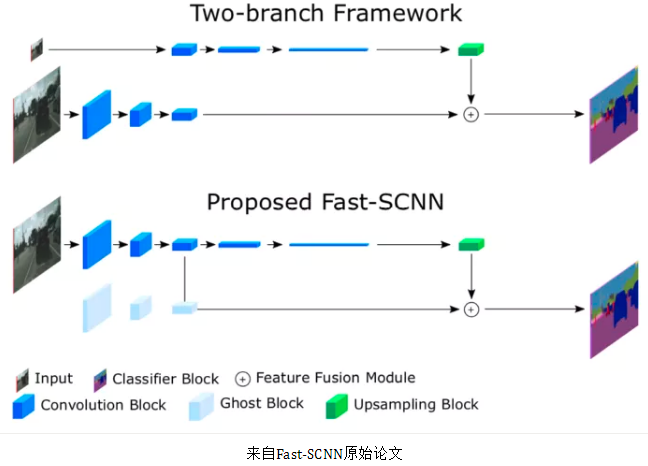在这个模块中，两个输入相加以更好地表示分割。第一个是从学习下采样模块中提取的高级特征，这个学习下采样模块先进行point-wise卷积，再加入到第二个输入中。这里在point-wise卷积的最后没有进行激活。

`ff_layer1 = conv_block(lds_layer, 'conv', 128, (1,1), padding='same', strides= (1,1), relu=False)`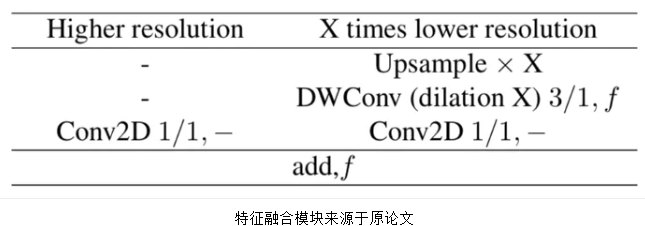这是使用TF.Keras实现的低分辨率操作：

```ff_layer2 = tf.keras.layers.UpSampling2D((4, 4))(gfe_layer)
ff_layer2 = tf.keras.layers.DepthwiseConv2D(128, strides=(1, 1), depth_multiplier=1, padding='same')(ff_layer2)
ff_layer2 = tf.keras.layers.BatchNormalization()(ff_layer2)
ff_layer2 = tf.keras.activations.relu(ff_layer2)
ff_layer2 = tf.keras.layers.Conv2D(128, 1, 1, padding='same', activation=None)(ff_layer2)```

```ff_final = tf.keras.layers.add([ff_layer1, ff_layer2])
ff_final = tf.keras.layers.BatchNormalization()(ff_final)
ff_final = tf.keras.activations.relu(ff_final)```

4. 分类器

```classifier = tf.keras.layers.SeparableConv2D(128, (3, 3), padding='same', strides = (1, 1), name = 'DSConv1_classifier')(ff_final)
classifier = tf.keras.layers.BatchNormalization()(classifier)
classifier = tf.keras.activations.relu(classifier)

classifier = tf.keras.layers.SeparableConv2D(128, (3, 3), padding='same', strides = (1, 1), name = 'DSConv2_classifier')(classifier)
classifier = tf.keras.layers.BatchNormalization()(classifier)
classifier = tf.keras.activations.relu(classifier)

classifier = conv_block(classifier, 'conv', 19, (1, 1), strides=(1, 1), padding='same', relu=True)

classifier = tf.keras.layers.Dropout(0.3)(classifier)

classifier = tf.keras.layers.UpSampling2D((8, 8))(classifier)
classifier = tf.keras.activations.softmax(classifier)```

`fast_scnn = tf.keras.Model(inputs = input_layer , outputs = classifier, name = 'Fast_SCNN')`

```optimizer = tf.keras.optimizers.SGD(momentum=0.9, lr=0.045)
fast_scnn.compile(loss='categorical_crossentropy', optimizer=optimizer, metrics=['accuracy'])```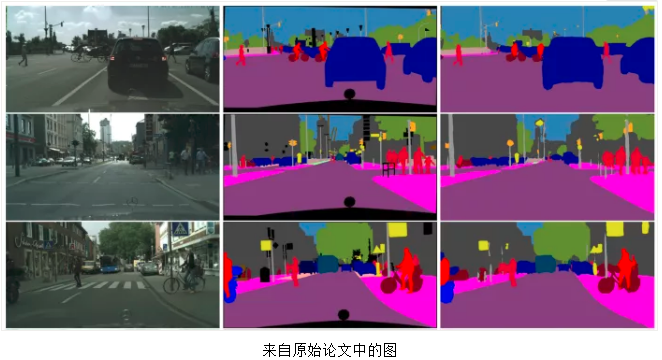500字Xavier1596265944

• 8

获得赞
• 4

发布的文章
• 0

答辩的项目